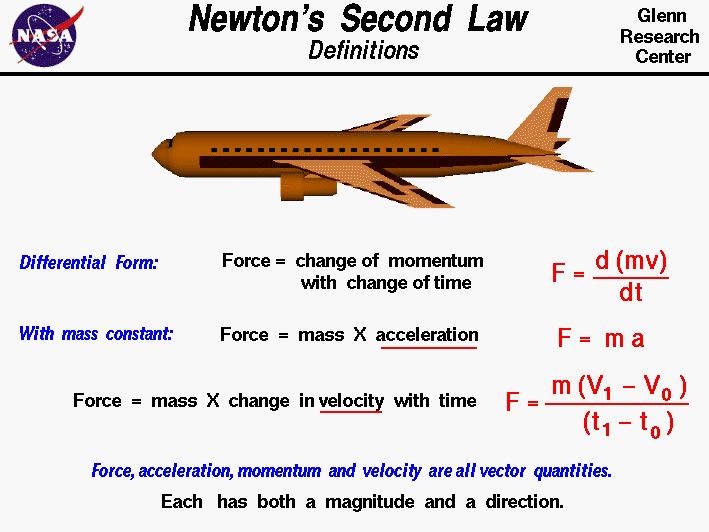+ Text Only Site
+ Non-Flash Version
+ Contact GlennSir Isaac Newton first presented his three laws of motion in the "Principia Mathematica Philosophiae Naturalis" in 1686. His second law defines a force to be equal to the differential change in momentum per unit time as described by the calculus of mathematics, which Newton also developed. The momentum is defined to be the mass of an object m times its velocity v. So the differential equation for force F is: F = d(m * v) / dt If the mass is a constant and using the definition of acceleration a as the change in velocity with time, the second law reduces to the more familiar product of a mass and an acceleration: F = m * a Since acceleration is a change in velocity with a change in time t, we can also write this equation in the third form shown on the slide: F = m * (v1 - v0) / (t1 - t0) The important fact is that a force will cause a change in velocity; and likewise, a change in velocity will generate a force. The equation works both ways. The velocity, force, acceleration, and momentum have both a magnitude and a direction associated with them. Scientists and mathematicians call this a vector quantity. The equations shown here are actually vector equations and can be applied in each of the component directions. The motion of an aircraft resulting from aerodynamic forces and the aircraft weight and thrust can be computed by using the second law of motion. Activities: Guided Tours Navigation ..Beginner's Guide Home Page+ Inspector General Hotline + Equal Employment Opportunity Data Posted Pursuant to the No Fear Act + Budgets, Strategic Plans and Accountability Reports + Freedom of Information Act + The President's Management Agenda + NASA Privacy Statement, Disclaimer, and Accessibility CertificationEditor: Tom Benson NASA Official: Tom Benson Last Updated: May 07 2021 + Contact Glenn